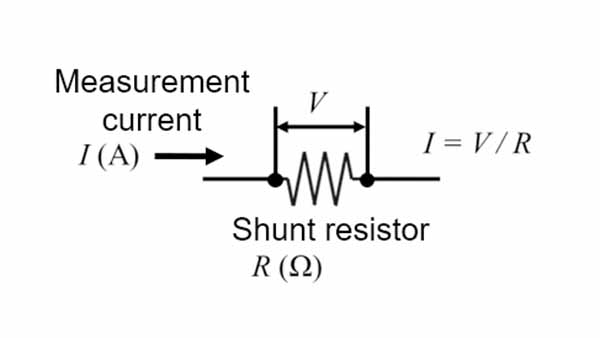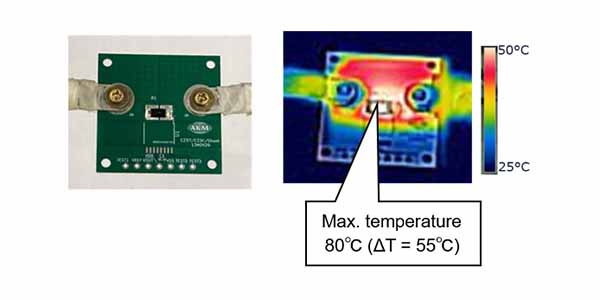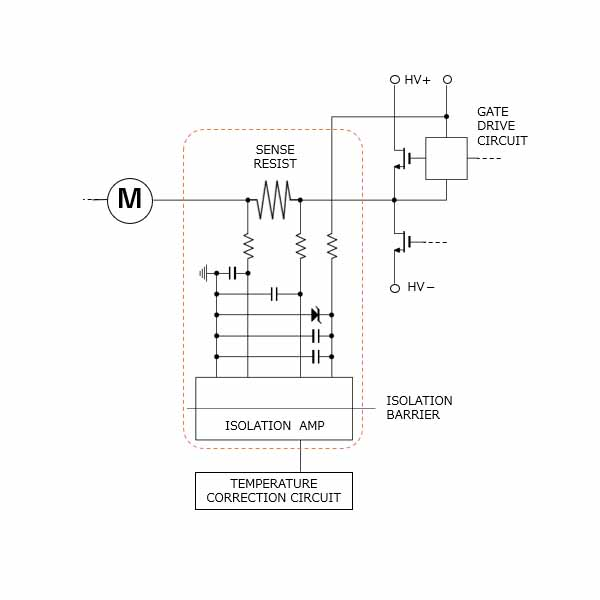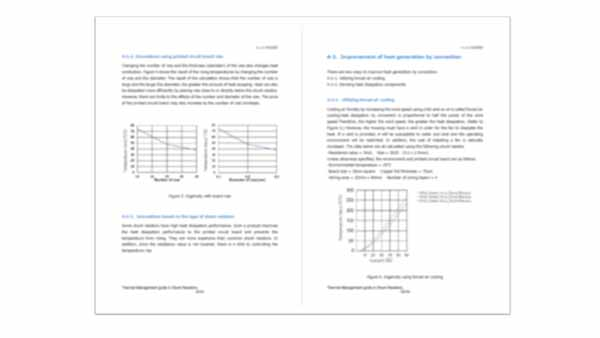# Shunt Resistor Current Measurement and Heat Generation

Current Sensors

Understand the principles of current sensing and heat generation using shunt resistors. This will help you understand how shunt current sensors generate heat and how to counteract it. You will learn the following

• Measuring Principle of Shunt Resistors and the Mechanism of Heat Generation
• Impact of Shunt Resistors Heat Generation and the Countermeasures for Heat Dissipation
• Low heat generation Current Sensing Methods

This page describes series-connected shunt resistors, such as those used primarily in current sensing.　We can also use shunt resistors in applications such as ammeters by connecting them in parallel. We can extend the measurement range by shunt current measurement.

## 1. Heat Generation in Shunt Resistor and its Principle

For industrial equipment such as motors and inverters, the monitoring of current is essential in terms of safety, performance, and efficiency. One type of current detection method is a shunt resistor.

As illustrated in Figure 1, we supply the current that we aim to measure through a shunt resistor with known resistance. We then measure the voltage across this shunt resistor. Using Ohm's law (V=IR), we can calculate the value of the supplied current. This is the most popular current detection method because of its simple principle and ease of use.

Just like a normal resistor, the shunt resistor also produces heat when it receives current. The calorific value is proportional to the square of the amperage and the resistance value according to Joule's law P=I2R.Figure 1. Principle of current detection by shunt resistor

## 2. Calorific value of shunt resistors

As explained, a shunt resistor generates heat based on its mechanism. Then, how much heat does a shunt resistor actually generate?

See Figure 2. The surface temperature of a shunt resistor was measured under the conditions shown at the righthand side. We found that the temperature rose to about 80℃ at the highest point. Its temperature increment is 55℃.

Since the temperature increment is proportional to the calorific value, it will be doubled if the resistance value is doubled, and will be quadrupled if the current value is doubled. Therefore, a shunt resistor is not suitable for measuring large currents. Generally, the magnitude of the current that can be used without any concern about heat generation is said to be around 10Arms.

Temperature rise can be controlled by reducing the resistance value of the shunt value provided that the same amperage is supplied. However, if the resistance value decreases, the voltage (V=IR) across the shunt resistor also decreases. The sensing voltage of the shunt register needs to be at a level that satisfies a sufficient S/N ratio in the subsequent signal processing. Therefore, it is not desirable to reduce the resistance value only for reducing heat generation.

Experimental conditions

・Shunt resistance = 5mΩ 　・Size = 6432 (6.4 x 3.2mm)
・Amount of current = 20A
・Room temperature (25°C) under natural air coolingFigure 2. Thermal image of shunt resistor

## 3. Impact of heat generation

As explained, it is difficult to reduce heat generation by lowering the resistance value due to the trade-off between the heat generation of the shunt resistor and the S/N ratio. So what can go wrong if the shunt resistor generates heat? There are two main problems.

1. Cost increase and parts size increase

Electronic components such as shunt resistors may be damaged due to excessive heat generation. For this reason, electronic components are rated and must be used safely with a margin. Generally, the larger the rating, the higher the cost and the larger the size.

Printed Circuit Boards(PCB) used in industrial equipment such as a motor or inverter contain a variety of components. In recent years, due to the integration at factories and other factors, these printed circuit boards have become smaller in size. In other words, many components are packed within a small printed circuit board.

Therefore, the ambient temperature of other electronic components rises due to the heat generated by the shunt resistor. As a result, it is necessary to select other components with large ratings such as the operating environment temperature, thereby increasing the entire system cost and hindering integration/miniaturization.

2. Increasing complexity of circuits and the number of parts

The resistance value of the shunt resistor fluctuates depending on the temperature as well as the normal resistance. Since the detected voltage is proportional to the resistance value of the shunt resistor, if the resistance value changes due to the temperature rise caused by heat generation, a deviation occurs in the calculated current value.

Therefore, in order to accurately detect the current with the shunt resistor, a temperature correction circuit is required to compensate for the temperature change of the shunt resistor. This makes the circuit more complex and hinders miniaturization by increasing the number of parts.

In this way, heat generation of the shunt resistor has a significant impact on the entire system.Figure 3. Circuit diagram when using a shunt resistor

## Want to understand and tackle thermal management ? Our guide, available for download here, provides clear solutions.

While electronic devices provide advanced performance, they also generate a large amount of heat within them. If this is not managed, it can not only shorten the life of the device, but also degrade its performance. To learn more, please download and read the specialized technical document we have prepared. Here, heat generation and its countermeasures are explained in depth.

Thermal Management guide in Shunt Resistors
English (16Pages PDF: 684KB)

This document describes in detail

-Mechanism of heat generation.
-How to control heat generation in shunt resistors.
-Heat dissipation in system.

and morePlease fill in the following form and send it to us.